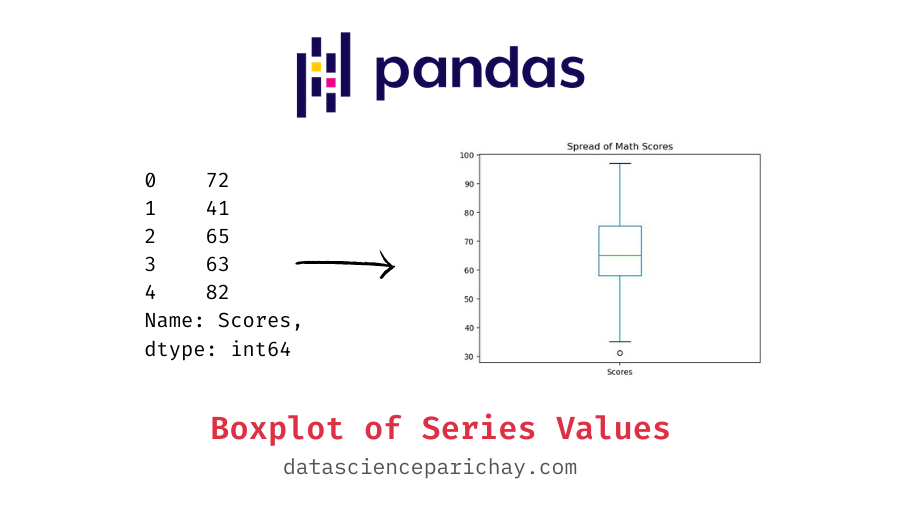# Create a Boxplot from Pandas Series Values

Boxplots are quite useful to visualize the spread in the data. They are particularly helpful in spotting outliers in the data. In this tutorial, we will look at how to create a boxplot from the values of a pandas series.

To plot a pandas series, you can use the pandas series `plot()` function. It plots a line chart of the series values by default but you can specify the type of chart to plot using the `kind` parameter. To create a boxplot, pass `'box'` to the `kind` paramter. The following is the syntax:

```# boxplot using pandas series plot()
s.plot(kind='box')```

Here, s is the pandas series you want to plot. The pandas series `plot()` function returns a matplotlib axes object to which you can add additional formatting.

Let’s look at some examples of plotting a pandas series values on a boxplot. First, we’ll create a sample pandas series which we will be using throughout this tutorial.

```import pandas as pd

# scores in the Math class
math_scores = pd.Series(data=[72, 41, 65, 63, 82, 63, 51, 57, 39, 63,
62, 68, 52, 76, 62, 73, 72, 73, 71, 62,
76, 53, 71, 79, 77, 35, 65, 59, 58, 70,
73, 69, 59, 75, 73, 63, 65, 81, 46, 59,
53, 71, 79, 80, 60, 60, 31, 40, 73, 75,
68, 58, 81, 65, 55, 62, 82, 47, 85, 62,
39, 77, 82, 78, 57, 58, 72, 75, 65, 68,
86, 49, 39, 64, 54, 68, 85, 77, 62, 53,
52, 76, 80, 84, 69, 61, 69, 65, 89, 97,
71, 61, 77, 40, 83, 52, 78, 54, 64, 58],
name='Scores')

Output:

```0    72
1    41
2    65
3    63
4    82
Name: Scores, dtype: int64```

You can see the top five values of the series object above. We now have a pandas series containing the scores of students in a Math class.

To create a boxplot from the series values we’ll pass `kind='box'` to the pandas series `plot()` function. For example, let’s see its usage on the “math_scores” series created above.

`math_scores.plot(kind='box')`

Output:

📚 Data Science Programs By Skill Level

Introductory

Intermediate ⭐⭐⭐

🔎 Find Data Science Programs 👨‍💻 111,889 already enrolled

Disclaimer: Data Science Parichay is reader supported. When you purchase a course through a link on this site, we may earn a small commission at no additional cost to you. Earned commissions help support this website and its team of writers.

The values within the edges of the boxplot depict values from the Q1 to the Q3 quartile of the series. The boxplot also shows the median value (Q2) of the series with a line inside the box. The whiskers extend from the box edges to no more than 1.5 * IQR (Inter Quartile Range = Q3 – Q1), values beyond the whiskers are depicted as dots representing the outliers.

In the above chart the green line depicting the median is around 65. Also notice that we have one outlier on the lower end of the scores represented by a dot.

You can also customize the formatting of the chart. For example, you can add the axes labels, chart title, etc. Since the returned plot is a matplotlib axes object, you can apply any formatting that would work with matplotlib charts. Let’s go ahead and add a title to our plot.

```# create the boxplot
ax = math_scores.plot(kind='box')
# set the title

Output:

For more on the pandas series plot() function, refer to its documentation.

With this, we come to the end of this tutorial. The code examples and results presented in this tutorial have been implemented in a Jupyter Notebook with a python (version 3.8.3) kernel having pandas version 1.0.5

•